# 使用原生js通过缓动函数实现抽奖转盘动画前端开发工程师 @ bigo# 前言

## 什么是缓动函数

### 线性运动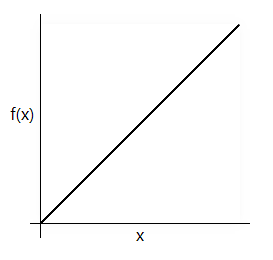### 缓入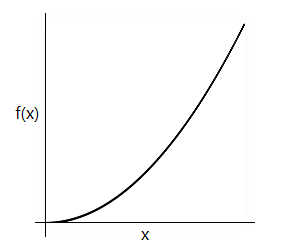### 缓出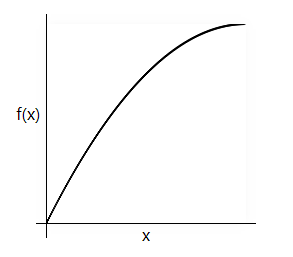### 缓入缓出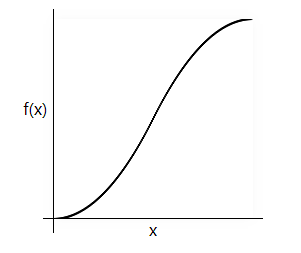``````// 原版
function QuadIn(t, b, c, d) {
return c * (t /= d) * t + b;
}

// t:缓动开始时间
// b:缓动开始位置
// c:缓动移动的距离
// d:缓动持续的时间

// 结果值为当前位置值

``````// 修改版
function QuadIn(p) {
return p * p;
}

// p: 当前已执行的时间 / 总时间

// 结果值需要与总移动距离相乘才获得当前位置

## 使用css实现缓动函数

### transition

``````.el {
transition-timing-function: ease-in-out;
}

### animation

``````.el {
animation-timing-function: ease-in-out;
}

## 使用三次贝塞尔曲线定义缓动函数

css的动画属性除了使用缓动函数的名称来定义之外，还可以通过三次贝塞尔曲线函数cubic-bezier()定义。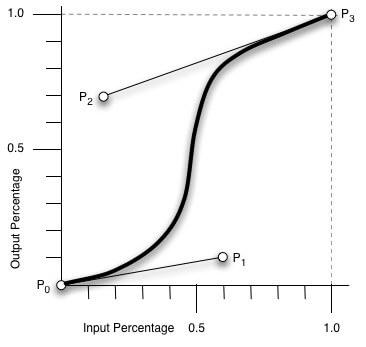``````.el {
transition-timing-function: cubic-bezier(0.42, 0, 0.58, 1);
animation-timing-function: cubic-bezier(0.42, 0, 0.58, 1);
}

## 利用原生js实现缓动函数

### 动画循环函数

• setTimeout实现
``````const rAF =  (callback) => {
return window.setTimeout(callback, 1000 / 60)
}
const amination = () => {

// 退出循环条件
if () {
return
}
rAF(amination);
}

rAF(amination);

• setInterval实现
``````const rAF =  (callback) => {
return window.setInterval(callback, 1000 / 60)
}

const amination = () => {

// 退出循环条件
if () {
return window.clearInterval(this.timer);
}
}

this.timer = rAF(amination);

• 使用浏览器提供的`requestAnimationFrame`方法
``````const amination = () => {

// 退出循环条件
if () {
return
}
window.requestAnimationFrame(amination);
};

window.requestAnimationFrame(amination);

### 转盘运动过程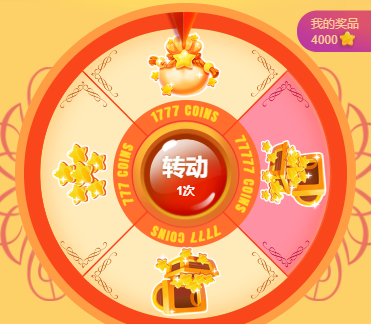#### 加速阶段

``````// 缓入函数
function eaeIsn(t, b, c, d) {
if (t >= d) t = d;
return c * (t /= d) * t + b;
}

// 开始时间
const startTime = Date.now();

// 最大速度，将每帧旋转的角度为看做速率
const maxSpeed = 20;

// 加速持续时间
const holdTime = 3000;

// 时间段的起点
const timeStartPoint = 0;

// 旋转角度
this.deg = 0;

function animation() {
// 当前使用的时间段
const currentTime = Date.now() - startTime;

// 获取当前帧的速度
const curSpeed = easeIn(currentTime, timeStartPoint, maxSpeed, holdTime);

// 旋转角度
this.deg += curSpeed;

// 优化结果值，取360度的余数结果即为当前位置
this.deg = this.deg % 360;

window.requestAnimationFrame(animation)
}

window.requestAnimationFrame(animation);

#### 减速阶段

``````// 缓入函数
function eaeIsn(t, b, c, d) {
if (t >= d) t = d;
return c * (t /= d) * t + b;
}

// 缓出函数
function easeOut (t, b, c, d) {
if (t >= d) t = d;
return -c * (t /= d) * (t - 2) + b;
}

// 开始时间
const startTime = Date.now();

// 最大速度
const maxSpeed = 20;

// 加速持续时间
const startHoldTime = 2000;

// 时间段的起点
const timeStartPoint = 0;

// 减速持续时间
const endHoldTime = 3000;

// 旋转角度
this.deg = 0;

// 停止区域索引
this.stopIndex = 0;

// 动画循环总次数，用来计算fps
this.progress = 0;

// 减速开始时间
this.endTime = 0;

// 每个奖品所占的角度
this.prizeDeg = 45;

function animation() {
// 当前使用的时间段
const currentTime = Date.now() - startTime;

// 获取当前帧的速度
const curSpeed = easeIn(currentTime, timeStartPoint, maxSpeed, holdTime);

// 旋转角度
this.deg += curSpeed;

// 优化结果值，取360度的余数结果即为当前位置
this.deg = this.deg % 360;

// 检测到stopIndex有值，此时知道抽到的奖品区间范围，开始实行减速，计算减速总路程
if (this.stopIndex > 0) {

// 计算屏幕刷新帧率
const fps = currentTime / this.progress;
this.endTime = Date.now();

// 开始减速时所处的位置
this.stopDeg = this.deg;
let i = 0;
while(++i) {
// 结合开始减速时所处的位置和结束时所处的位置计算旋转总路程
const endDeg = 360 * i - this.stopIndex * this.prizeDeg - this.stopDeg;

// 计算刚开始第一帧旋转的角度，也就是初始速度
const curSpeed = easeOut(fps, stopDeg, endDeg, endHoldTime) - this.stopDeg;

// 当初始速度与当前旋转最大速度相等，即可获取总共需要旋转的角度
if (curSpeed >= maxSpeed) {
this.endDeg = endDeg;
break;
}
}

// 开始减速
return slowDown();
}

window.requestAnimationFrame(animation)
}

function slowDown() {
window.requestAnimationFrame(function() {
const currentTime = Date.now() - this.endTime;

// 减速完成
if (currentTime >= endHoldTime) {
return;
}

// 缓出减速
this.deg = easeOut(currentTime, this.stopDeg, this.endDeg, endHoldTime) % 360;
this.slowDown();
})
}

window.requestAnimationFrame(animation);

### 性能优化

``````.el {
will-change: transform;
}